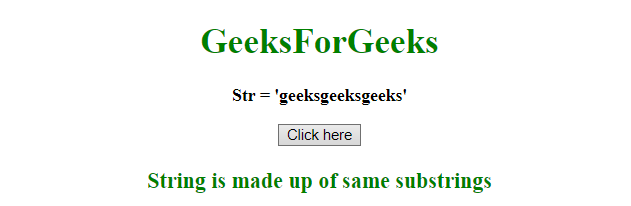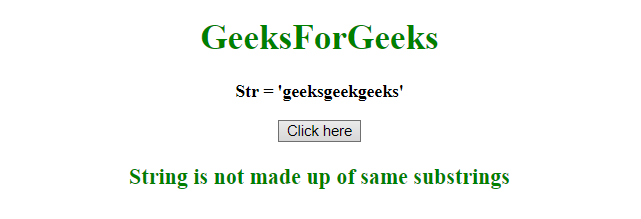# How to check a string is entirely made up of the same substring in JavaScript ?

Given a string and the task is to determine whether the string is made up of the same substrings or not.

Approach:

• Initialize a string to the variable.
• Use test() method to test a pattern in a string.
• The test() method returns true if match is found, otherwise it returns false.

Example 1: This example checks for string geeksgeeksgeeks which is made up of same substring, so it returns True.

 `  ` `<``html``>  ` `    ``<``head``>  ` `        ``<``title``>  ` `            ``How to check a string is entirely ` `            ``made of same substring ? ` `        `` ` `    ``  ` `     `  `    ``<``body` `style` `= ``"text-align:center;"``>  ` ` `  `        ``<``h1` `style` `= ``"color:green;"` `>  ` `            ``GeeksForGeeks  ` `        ``  ` ` `  `        ``<``p` `id` `= ``"GFG_UP"` `style` `= ` `            ``"font-size: 16px; font-weight: bold;"``>      ` `        `` ` `         `  `        ``<``button` `onclick` `= ``"gfg_Run()"``>  ` `            ``Click here ` `        `` ` `         `  `        ``<``p` `id` `= ``"GFG_DOWN"` `style` `= ` `            ``"color:green; font-size: 20px; font-weight: bold;"``> ` `        `` ` `         `  `        ``<``script``> ` `            ``var el_up = document.getElementById("GFG_UP"); ` `            ``var el_down = document.getElementById("GFG_DOWN"); ` `         `  `            ``var str = "geeksgeeksgeeks"; ` `         `  `            ``el_up.innerHTML = "Str = '" + str + "'"; ` `            ``function check(str) { ` `                ``return /^(.+)\1+\$/.test(str) ` `            ``} ` `             `  `            ``function gfg_Run() { ` `                ``ans = "String is not made up of same substrings"; ` `                ``if (check(str)) { ` `                    ``ans = "String is made up of same substrings";  ` `                ``} ` `                ``el_down.innerHTML = ans; ` `            ``}          ` `        ``  ` `    ``  ` `                     `

Output:

• Before clicking on the button:• After clicking on the button:Example 2: This example checks for string geeksgeekgeeks which is not made up of same substrings so it returns False.

 `  ` `<``html``>  ` `    ``<``head``>  ` `        ``<``title``>  ` `            ``How to check a string is entirely ` `            ``made of same substring ? ` `        `` ` `    ``  ` `     `  `    ``<``body` `style` `= ``"text-align:center;"``>  ` ` `  `        ``<``h1` `style` `= ``"color:green;"` `>  ` `            ``GeeksForGeeks  ` `        ``  ` ` `  `        ``<``p` `id` `= ``"GFG_UP"` `style` `= ` `            ``"font-size: 16px; font-weight: bold;"``>      ` `        `` ` `         `  `        ``<``button` `onclick` `= ``"gfg_Run()"``>  ` `            ``Click here ` `        `` ` `         `  `        ``<``p` `id` `= ``"GFG_DOWN"` `style` `= ` `            ``"color:green; font-size: 20px; font-weight: bold;"``> ` `        `` ` `         `  `        ``<``script``> ` `            ``var el_up = document.getElementById("GFG_UP"); ` `            ``var el_down = document.getElementById("GFG_DOWN"); ` `         `  `            ``var str = "geeksgeekgeeks"; ` `         `  `            ``el_up.innerHTML = "Str = '" + str + "'"; ` `            ``function check(str) { ` `                ``return /^(.+)\1+\$/.test(str) ` `            ``} ` `             `  `            ``function gfg_Run() { ` `                ``ans = "String is not made up of same substrings"; ` `                ``if (check(str)) { ` `                    ``ans = "String is made up of same substrings";  ` `                ``} ` `                ``el_down.innerHTML = ans; ` `            ``}          ` `        ``  ` `    ``  ` `                     `

Output:

• Before clicking on the button:• After clicking on the button:My Personal Notes arrow_drop_upCheck out this Author's contributed articles.

If you like GeeksforGeeks and would like to contribute, you can also write an article using contribute.geeksforgeeks.org or mail your article to contribute@geeksforgeeks.org. See your article appearing on the GeeksforGeeks main page and help other Geeks.

Please Improve this article if you find anything incorrect by clicking on the "Improve Article" button below.